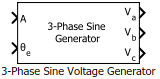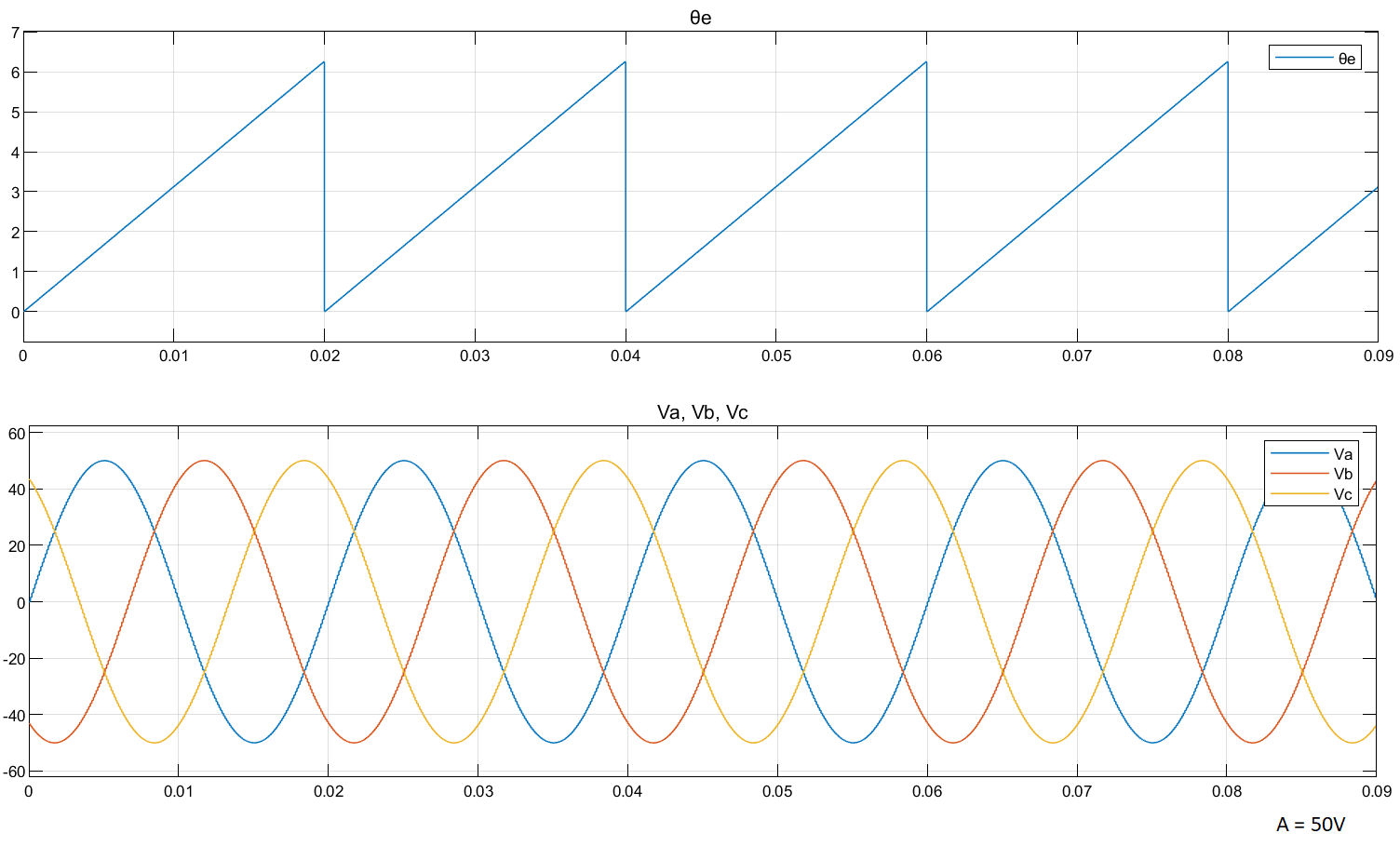3-Phase Sine Voltage Generator

Generate balanced three-phase sinusoidal signals

• Library:
• Motor Control Blockset / Controls / Math Transforms

•Description

The 3-Phase Sine Voltage Generator block generates balanced, three-phase sinusoidal signals using signal amplitude and position inputs.

The block uses the lookup table approach for optimized code-execution. You can specify the number of lookup table points in the Number of data points for lookup table parameter.

The following image shows a plot of position input and three-phase sinusoidal output signals against time.Equations

The following equations describe how the block computes balanced, three-phase sinusoidal signals.

• ${V}_{a}=A×\mathrm{sin}\omega t$

• ${V}_{b}=A×\mathrm{sin}\left(\omega t-\frac{2\pi }{3}\right)$

• ${V}_{c}=A×\mathrm{sin}\left(\omega t-\frac{4\pi }{3}\right)$

where:

• $A$ is the reference voltage amplitude (volts).

• $\omega$ is the frequency of the reference voltage position input signal (θe) (radians/ sec).

• $t$ is the time (seconds).

Ports

Input

expand all

Maximum amplitude of the reference voltage signal.

Data Types: single | double | fixed point

Position or phase value of the reference voltage signal.

Data Types: single | double | fixed point

Output

expand all

Balanced, three-phase voltage signal component along the a-axis of the abc reference frame.

Data Types: single | double | fixed point

Balanced, three-phase voltage signal component along the b-axis of the abc reference frame.

Data Types: single | double | fixed point

Balanced, three-phase voltage signal component along the c-axis of the abc reference frame.

Data Types: single | double | fixed point

Parameters

expand all

Unit of the reference voltage position that you provide as input.

Size of the lookup table.